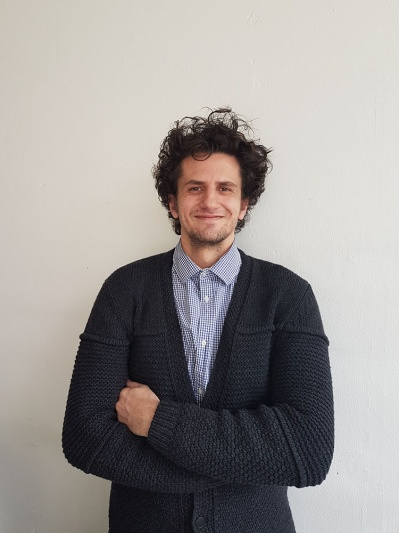# Soutenance de thèse Dimche KostadinovM. Dimche Kostadinov soutiendra en anglais, en vue de l'obtention du grade de docteur ès sciences mention informatique de la Faculté des sciences de l'Université de Genève, sa thèse intitulée: Nonlinear Transform Learning: Model, Applications and Algorithms Date: Jeudi 6 décembre 2018 à 14h30 Lieu: CUI / Battelle bâtiment D, Amphithéâtre

Jury:

• Prof. Svyatoslav Voloshynovskiy (Directeur, Université de Genève)
• Prof. Karen Egiazarian (Université de Tampere, Finlande)
• Prof. Teddy Furon  (INRIA, Rennes, France)
• Prof. Sylvain Sardy (Université de Genève)
• Prof. Stéphane Marchand-Maillet (Université de Genève)

Résumé:

Modeling of nonlinearities is essential for many real-world problems, where its treatment plays a central role and impacts not only the quality of the solution but also the computational complexity. Its high prevalence impacts on a variety of applications, including active content fingerprinting, image restoration, supervised and unsupervised discriminative representation learning for image recognition tasks and clustering.

In this thesis, we introduce and study a novel generalized nonlinear transform model. In particular, our main focus and core element is on the nonlinear transform that is expressible by a two-step operation consisting of linear mapping, which is followed by element-wise nonlinearity. To that end, depending on the considered application, we unfold probabilistic interpretations, propose generalizations, extensions and take into account special cases. An approximation to the empirical likelihood of our nonlinear transform model provides a learning objective, where we not only identify and analyze the corresponding trade-offs, but we give information-theoretic as well as empirical risk connections considering the addressed objectives in the respective problem formulations. We introduce a generalization that extends an integrated maximum marginal principle over the approximation to the empirical likelihood, which allows us to address the optimal parameter estimation. In this scope, depending on the modeled assumptions w.r.t. an application objective, the implementation of the maximum marginal principle enables us to efficiently estimate the model parameters where we propose an approximate and exact closed form solutions as well as present iterative algorithms with convergence guarantees.

Numerical experiments empirically validate the nonlinear transform model, the learning principle, and the algorithms for active content fingerprinting, image denoising, estimation of robust and discriminative nonlinear transform representation for image recognition tasks and our clustering method that is preformed in the nonlinear transform domain. At the moment of thesis preparation our numerical results demonstrate advantages in comparison to the state-of-the-art methods of the corresponding category, regarding the learning time, the run time and the quality of the solution.